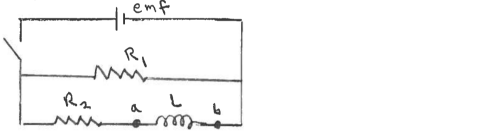# Problem: Consider the circuit shown in the sketch. The battery emf is 48.0 V,  R1 = 16.0 Ω, and R2 = 6.00 Ω. Initially no currents are flowing. Then the switch is closed. After the switch has been closed a long time, what is the voltage  vab across the inductor?

###### Problem Details

Consider the circuit shown in the sketch. The battery emf is 48.0 V,  R1 = 16.0 Ω, and R2 = 6.00 Ω. Initially no currents are flowing. Then the switch is closed. After the switch has been closed a long time, what is the voltage  vab across the inductor?